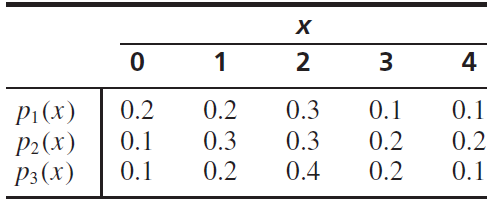×
Get Full Access to Statistics For Engineers And Scientists - 4 Edition - Chapter 2.4 - Problem 4e
Get Full Access to Statistics For Engineers And Scientists - 4 Edition - Chapter 2.4 - Problem 4e

×

# Let X represent the number of tires with low air pressureISBN: 9780073401331 38

## Solution for problem 4E Chapter 2.4

Statistics for Engineers and Scientists | 4th Edition

• Textbook Solutions
• 2901 Step-by-step solutions solved by professors and subject experts
• Get 24/7 help from StudySoup virtual teaching assistantsStatistics for Engineers and Scientists | 4th Edition

4 5 1 339 Reviews
28
5
Problem 4E

Let X represent the number of tires with low air pressure on a randomly chosen car.

a. Which of the three functions below is a possible probability mass function of X? Explain.b. For the possible probability mass function, compute μX and.

Step-by-Step Solution:

Solution:

Step 1 of 3:

Let X denote the number of tires with low air pressure on a randomly chosen car.

 X 0 1 2 3 40.2 0.2 0.3 0.1 0.1(x) 0.1 0.3 0.3 0.3 0.2(x) 0.1 0.2 0.2 0.4 0.1

We have to find

1. Which of given  three functions is a possible probability mass function of X.
2. For the possible mass function,we have to compute E(x) and V(X).

Step 2 of 3

Step 3 of 3

##### ISBN: 9780073401331

Unlock Textbook Solution